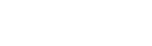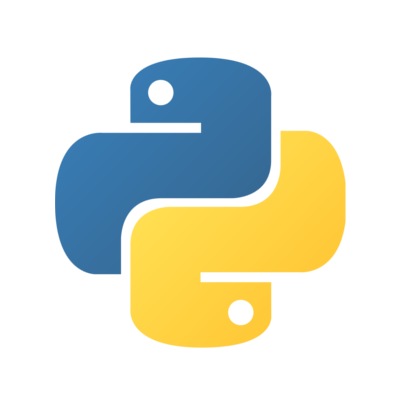#Fedora Developer Portal## Python

General-purpose, high-level programming language supporting multiple programming paradigms.

# Scientific Python Stack

There are lot of libraries for scientific computation and visualization available in Fedora. Well known and widely used is SciPy Stack which consists of

• Python, a general purpose object-oriented programming language
• NumPy, a Python library providing fast multidimensional arrays with vector operations
• SciPy, a Python library providing computational routines, for example numerical integration, various equation solvers and optimization
• matplotlib, a powerful Python library providing scientific visualization of publication quality
• IPython, an enhanced interactive Python interpreter
• pandas, a Python library providing data type for data series manipulation
• SymPy, a Python library for computer algebra support (i.e. symbolical computation)
• Jupyter Notebook, a web app that allows you to create and share live code, equations, visualizations and explanatory text

## NumPy

NumPy is a high performance Python library providing fast multidimensional arrays featuring vector operations.

NumPy can be installed in Fedora by typing:

``````\$ sudo dnf install python3-numpy
``````

What are vector operations? The ones which are applied on multiple values at once:

``````>>> import numpy as np
>>> A = np.array([[1, 2], [3, 4]])
>>> A
array([[1, 2],
[3, 4]])
>>> A+1
array([[2, 3],
[4, 5]])
>>> -1*A
array([[-1, -2],
[-3, -4]])
>>> A**2
array([[ 1,  4],
[ 9, 16]])
>>> A[0,:]
array([1, 2])
>>> A[0,:]=0
>>> A
array([[0, 0],
[3, 4]])
``````

NumPy also provides additional mathematical functions like `sin`, `cos`, `arcsin`, `exp`, `log`, `min`, `max`, `sum` and others.

``````>>> np.sin([0, np.pi/6, np.pi/2])
array([ 0. ,  0.5,  1. ])
>>> 2*np.arcsin([0, 1])
array([ 0.        ,  3.14159265])
``````

NumPy includes some handy shortcuts:

``````>>> np.zeros((3, 3))
array([[ 0.,  0.,  0.],
[ 0.,  0.,  0.],
[ 0.,  0.,  0.]])
>>> np.ones((3, 3))
array([[ 1.,  1.,  1.],
[ 1.,  1.,  1.],
[ 1.,  1.,  1.]])
>>> np.ones((3, 1))
array([[ 1.],
[ 1.],
[ 1.]])
>>> np.zeros_like(np.ones((1, 3)))
array([[ 0.,  0.,  0.]])
``````

## SciPy

SciPy is a Python library providing routines for basic and special mathematical functions, numerical integration, optimization, interpolation, Fourier transform, signal processing, routines for linear algebra, statistics and others. See a full SciPy reference.

To install it, type:

``````\$ sudo dnf install python3-scipy
``````

To compute, for example, the definite integral of 2x from 0 to 1 (which equals 1), type:

``````>>> import scipy.integrate
>>> scipy.integrate.quad(lambda x: 2*x, 0, 1)
(1.0, 1.1102230246251565e-14)
``````

## matplotlib

matplotlib is a graph plotting library producing publication-quality plots.

To install it, type:

``````\$ sudo dnf install python3-matplotlib
``````

Test it by typing:

``````>>> import matplotlib.pyplot as plt
>>> plt.ion()
>>> plt.plot([1, 2, 3], [10, 20, 30], 'ro--')
>>> plt.title("Hello, matplotlib!")
``````

You should see the plot already.

See lots of other examples at a showcases gallery:## IPython

IPython is a rich Python interpreter aiming at high-quality user experience for interactive computing and data visualization. Its main features are a tab completion, integration of commands for filesystem access, object introspection and others. IPython provides the kernel for the Jupyter project.

To install and run IPython, type:

``````\$ sudo dnf install python3-ipython
``````
``````\$ ipython3
Python 3.5.1 (default, Mar  4 2016, 15:21:15)

IPython 3.2.1 -- An enhanced Interactive Python.
?         -> Introduction and overview of IPython's features.
%quickref -> Quick reference.
help      -> Python's own help system.
object?   -> Details about 'object', use 'object??' for extra details.

In : print("Hello, world!")
Hello, world!
``````

## pandas

Pandas is a Python library implementing a data type to store data series and relevant routines:

``````\$ sudo dnf install python3-pandas
``````

Let us load famous Iris dataset, which is used a lot as an example in pattern recognition and machine learning literature.

``````import pandas as pd

iris_data_set_url = ("http://archive.ics.uci.edu/ml/"
"machine-learning-databases/iris/iris.data")

# pandas likes CSV files, it can read them from file or provided URL

# See!
print("Pandas DataFrame:")
print(my_first_pandas_DataFrame)

# Let us find a record with maximal value in the first column
print(my_first_pandas_DataFrame.max(0))
``````

See more on its usage in a pandas tutorial.

## SymPy

SymPy extends SciPy with symbolic computation capabilities, i.e. manipulation with algebraic variables, symbols and precise values.

First, install SymPy by running:

``````\$ sudo dnf install python3-sympy
``````

and then your journey to the realm of CAS (Computer Algebra System) can begin:

``````>>> import sympy
>>> sympy.sqrt(8)
2*sqrt(2)
>>> from sympy import symbols, exp, integrate, oo, diff
>>> x = symbols('x')
>>> integrate(exp(-x), (x, 0, +oo))
1
>>> integrate(exp(-x), (x, -oo, +oo))
oo
>>> diff(exp(-x))
-exp(-x)
``````

## Jupyter Notebook

Run and share live code via the browser; uses include: data cleaning and transformation, numerical simulation, statistical modeling, machine learning.

First install Jupyter Notebook:

``````\$ sudo dnf install notebook
``````

To start a notebook server run:

``````\$ jupyter notebook
``````

Next select the dropdown New then Python 3 to create a new notebook.NumPy, Pandas, SciPy and IPython are included with the install of Jupyter Notebook.